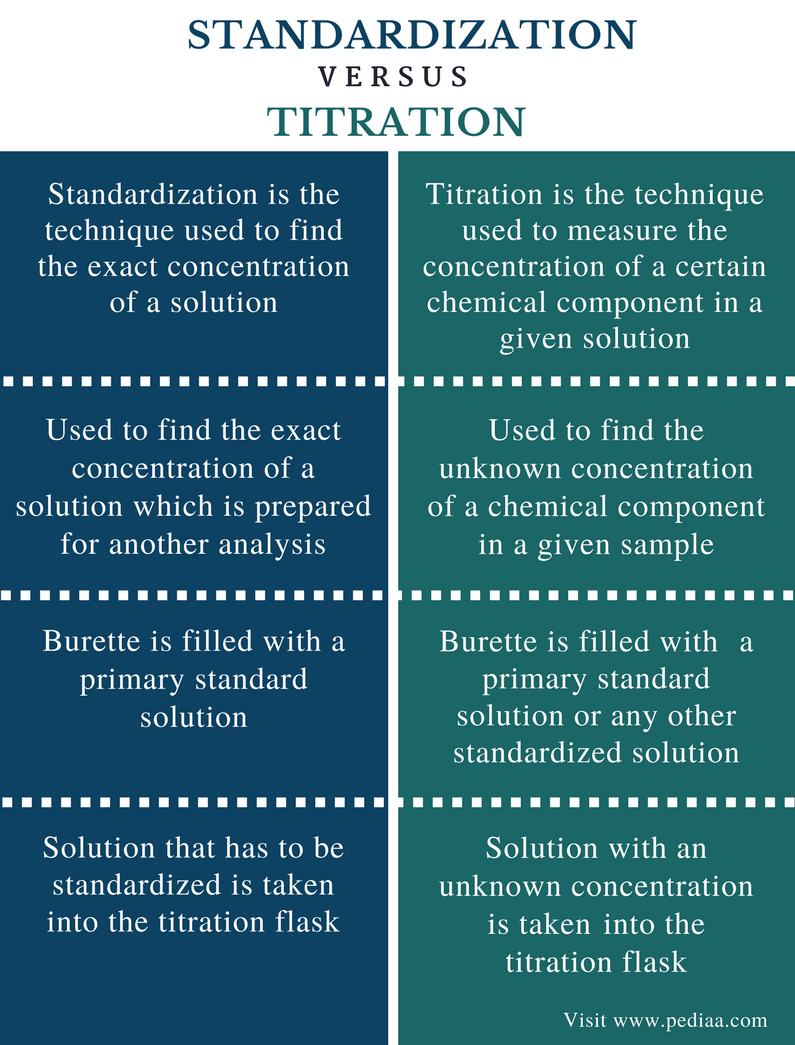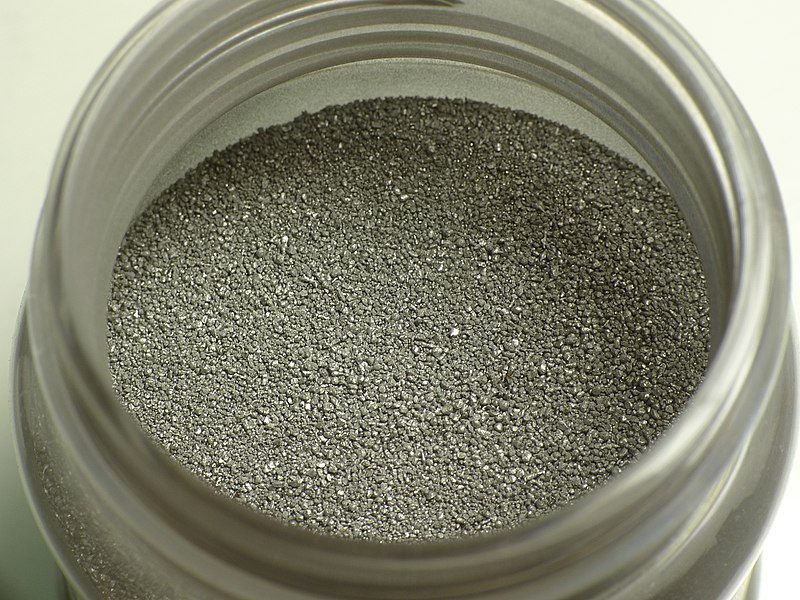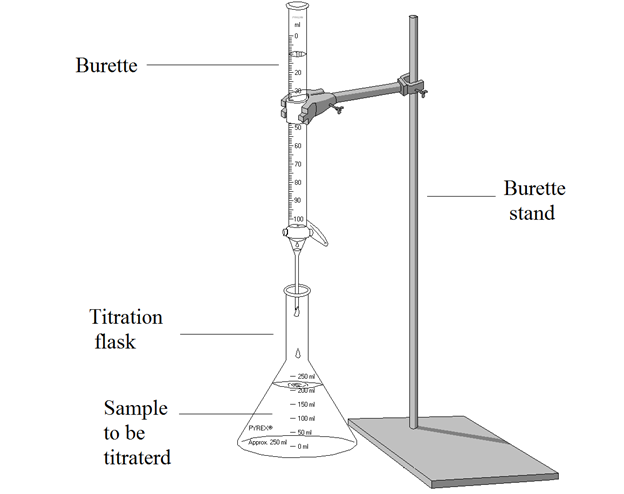# Difference Between Standardization and Titration

## Main Difference – Standardization vs Titration

Standardization and titration are two related chemical terms. Although they use the same technique to get a measurement, their applications are different from each other. Standardization is used to determine the exact concentration of a prepared solution. Titrations are used to determine the unknown concentrations of samples. Most often, standardization is also done as a titration. The main difference between standardization and titration is that standardization processes essentially uses primary standard solutions whereas titrations do not essentially use primary standard solutions.

### Key Areas Covered

1. What is Standardization

– Definition, Technique
2. What is Titration

– Definition, Technique
3. What is the Difference Between Standardization and Titration

– Comparison of Key Differences

Key Terms: End Point, Equivalence Point, Indicator, Primary Standard, Secondary Standard, Standardization, Titration## What is Standardization

Standardization is the technique used to find the exact concentration of a solution. The most commonly used technique for the standardization of a solution is titration. For a standardization process, a standard solution is required as a reference. Standard solutions can be found in two types as primary standard solutions and secondary standard solutions. For accurate standardizations, we use primary standard solutions. These solutions comprise of a high purity.Figure 1: Pure Iron Powder is a Primary Standard

When we make a solution using a solid chemical compound the final concentration of that solution may vary depending on several factors such as purity of the compound, instrumental errors, human errors, etc. For example, if we want to make a 1.0 molL-1 solution of EDTA, we can weigh the appropriate amount required for the preparation and dissolve it in a suitable volume of water. The required weight can be calculated using the data given on the bottle label. But this may not give the exact concentration that we need. Therefore, after the preparation of the solution, it should be standardized using a primary standard solution to find the exact concentration of the prepared solution.

## What is Titration

Titration is a chemical technique that is used to measure the concentration of a certain chemical component in a given solution. This is done using a solution which has a known concentration. A titration is done using a specific apparatus. This apparatus is given in the image below.Figure 2: Titration Apparatus

The burette is generally filled with a standard solution with a known concentration. If not, the solution in the burette should be standardized using a primary standard. The titration flask is filled with the sample that has the chemical component with unknown concentration. If the standardized solution (in burette) cannot act as a self-indicator, we should add a suitable indicator to the sample in the titration flask. Then, the standardized solution is added to the flask slowly until a color change is observed. The color change in the titration flask indicates the end point of the titration. Although it is not the exact point at which the titration ends, we can take it as the equivalence point since there is only a slight difference.

The burette reading can be used to find the amount of standard solution that reacted with the sample. Then by using chemical reactions and stoichiometric relationships, we can determine the concentration of the unknown.

## Difference Between Standardization and Titration

### Definition

Standardization: Standardization is the technique used to find the exact concentration of a solution.

Titration: Titration is the technique used to measure the concentration of a certain chemical component in a given solution.

### Application

Standardization: Standardization is used to find the exact concentration of a solution which is prepared for another analysis.

Titration: Titration is used to find the unknown concentration of a chemical component in a given sample.

### Solution in Burette

Standardization: For standardization, the burette is filled with a primary standard solution.

Titration: For titration, the burette is filled with either a primary standard solution or any other standardized solution.

Standardization: For standardization, the solution that has to be standardized is taken into the titration flask.

Titration: For titration, the solution that has the chemical component with an unknown concentration is taken.

### Conclusion

Standardizations are often done as titrations due to the ease of handling. Therefore they are related chemical terms. The main difference between standardization and titration is that standardization processes essentially use primary standard solutions whereas titrations do not essentially use primary standard solutions.

##### References:

1. “Titration.” Encyclopædia Britannica, Encyclopædia Britannica, inc., 11 Mar. 2014, Available here.
2. Eddy, Danny. Chemistry 104: Standardization of Acid and Base Solutions. Available here.
3. “Titration.” Chemistry LibreTexts, Libretexts, 24 July 2016, Available here.

##### Image Courtesy:

1. “Iron powder” By Anonimski – Own work (CC0) via Commons Wikimedia
2. “Titration Apparatus” By Ivan Akira – Own work (CC BY-SA 3.0) via Commons Wikimedia (Labeled)